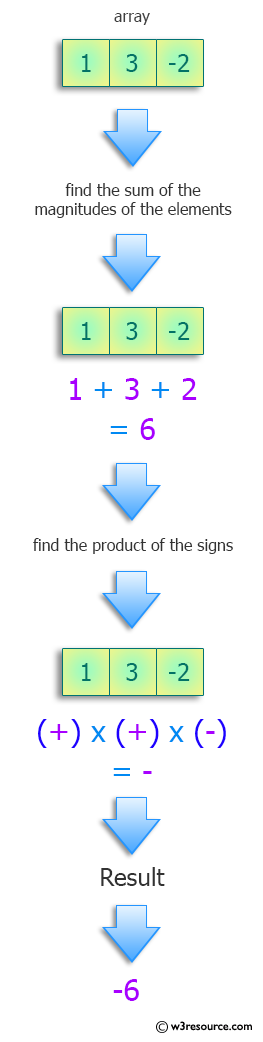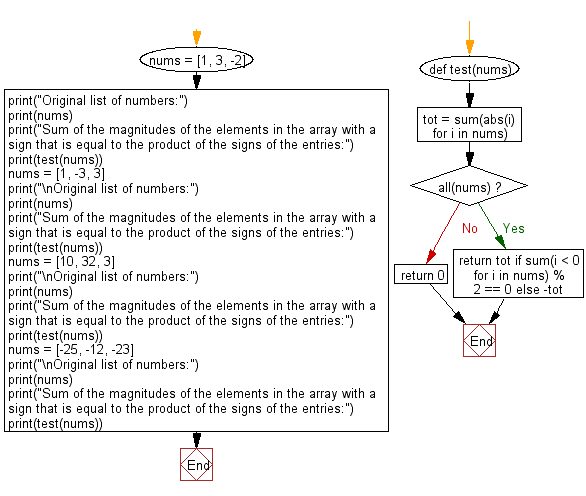﻿ Python: Sum of the magnitudes of the elements in the array with product signs - w3resource# Python: Sum of the magnitudes of the elements in the array with product signs

## Python Programming Puzzles: Exercise-57 with Solution

Write a Python program to find the sum of the magnitudes of the elements in the array with a sign that is equal to the product of the signs of the entries.

```Input:
[1, 3, -2]
Output:
-6

Input:
[1, -3, 3]
Output:
-7

Input:
[10, 32, 3]
Output:
45

Input:
[-25, -12, -23]
Output:
-60
```

Pictorial Presentation:Sample Solution:

Python Code:

``````def test(nums):
tot = sum(abs(i) for i in nums)
if all(nums):
return tot if sum(i < 0 for i in nums) % 2 == 0 else -tot
return 0

nums = [1, 3, -2]
print("Original list of numbers:")
print(nums)
print("Sum of the magnitudes of the elements in the array with a sign that is equal to the product of the signs of the entries:")
print(test(nums))
nums = [1, -3, 3]
print("\nOriginal list of numbers:")
print(nums)
print("Sum of the magnitudes of the elements in the array with a sign that is equal to the product of the signs of the entries:")
print(test(nums))
nums = [10, 32, 3]
print("\nOriginal list of numbers:")
print(nums)
print("Sum of the magnitudes of the elements in the array with a sign that is equal to the product of the signs of the entries:")
print(test(nums))
nums = [-25, -12, -23]
print("\nOriginal list of numbers:")
print(nums)
print("Sum of the magnitudes of the elements in the array with a sign that is equal to the product of the signs of the entries:")
print(test(nums))
``````

Sample Output:

```Original list of numbers:
[1, 3, -2]
Sum of the magnitudes of the elements in the array with a sign that is equal to the product of the signs of the entries:
-6

Original list of numbers:
[1, -3, 3]
Sum of the magnitudes of the elements in the array with a sign that is equal to the product of the signs of the entries:
-7

Original list of numbers:
[10, 32, 3]
Sum of the magnitudes of the elements in the array with a sign that is equal to the product of the signs of the entries:
45

Original list of numbers:
[-25, -12, -23]
Sum of the magnitudes of the elements in the array with a sign that is equal to the product of the signs of the entries:
-60
```

Flowchart:## Visualize Python code execution:

The following tool visualize what the computer is doing step-by-step as it executes the said program:

Python Code Editor :

Have another way to solve this solution? Contribute your code (and comments) through Disqus.

What is the difficulty level of this exercise?

Test your Programming skills with w3resource's quiz.

﻿

## Python: Tips of the Day

Clamps num within the inclusive range specified by the boundary values x and y:

Example:

```def tips_clamp_num(num,x,y):
return max(min(num, max(x, y)), min(x, y))
print(tips_clamp_num(2, 4, 6))
print(tips_clamp_num(1, -1, -6))
```

Output:

```4
-1
```